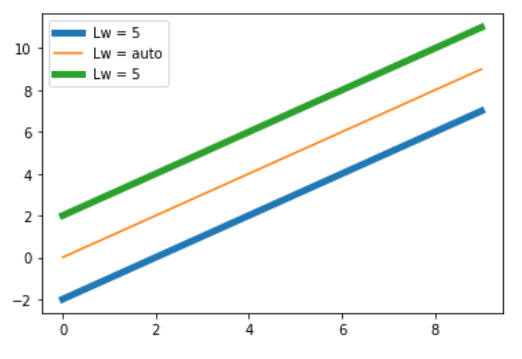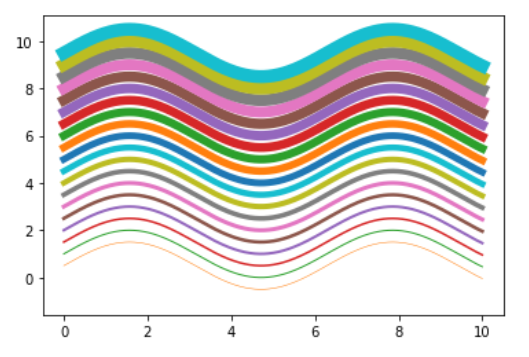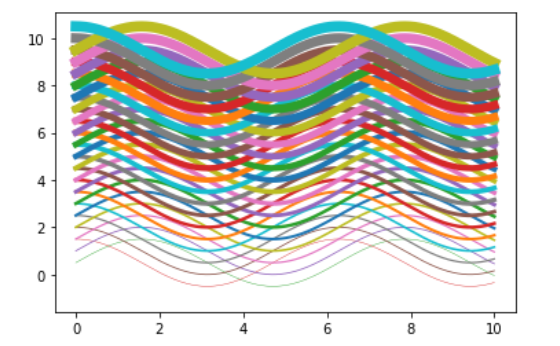Related Articles

# How to Change the Line Width of a Graph Plot in Matplotlib with Python?

• Last Updated : 12 Nov, 2020

Prerequisite : Matplotlib

In this article we will learn how to Change the Line Width of a Graph Plot in Matplotlib with Python. For that one must be familiar with the given concepts:

• Matplotlib : Matplotlib is a tremendous visualization library in Python for 2D plots of arrays. Matplotlib may be a multi-platform data visualization library built on NumPy arrays and designed to figure with the broader SciPy stack. It was introduced by John Hunter within the year 2002.
• Graph Plot : A plot is a graphical technique for representing a data set, usually as a graph showing the relationship between two or more variables.
• Line Width : The width of a line is known as line width. One can change the line width of a graph in matplotlib using a feature.

### Approach

• Import packages
• Import or create the data
• Draw a graph plot with a line
• Set the line width by using line-width feature ( lw can also be used as short form ).

Example 1:

## Python3

 `# importing packages``import` `matplotlib.pyplot as plt``import` `numpy as np`` ` `# create data``x_values ``=` `np.arange(``0``, ``10``)``y_values ``=` `np.arange(``0``, ``10``)`` ` `# Adjust the line widths``plt.plot(x_values, y_values ``-` `2``, linewidth``=``5``)``plt.plot(x_values, y_values)``plt.plot(x_values, y_values ``+` `2``, lw``=``5``)`` ` `# add legends and show``plt.legend([``'Lw = 5'``, ``'Lw = auto'``, ``'Lw = 5'``])``plt.show()`

Output :Example 2 :

## Python3

 `# importing packages``import` `matplotlib.pyplot as plt``import` `numpy as np`` ` `# create data``x_values ``=` `np.linspace(``0``, ``10``, ``1000``)``y_values ``=` `np.sin(x_values)`` ` `# Adjust the line widths``for` `i ``in` `range``(``20``):``    ``plt.plot(x_values, y_values ``+` `i``*``0.5``, lw``=``i``*``0.5``)``     ` `plt.show()`

Output :Example 3 :

## Python3

 `# importing packages``import` `matplotlib.pyplot as plt``import` `numpy as np`` ` `# create data``x_values ``=` `np.linspace(``0``, ``10``, ``1000``)`` ` `# Adjust the line widths``for` `i ``in` `range``(``20``):``    ``plt.plot(x_values, np.sin(x_values) ``+` `i``*``0.5``, lw``=``i``*``0.4``)``    ``plt.plot(x_values, np.cos(x_values) ``+` `i``*``0.5``, lw``=``i``*``0.4``)``     ` `plt.show()`

Output :Attention geek! Strengthen your foundations with the Python Programming Foundation Course and learn the basics.

To begin with, your interview preparations Enhance your Data Structures concepts with the Python DS Course. And to begin with your Machine Learning Journey, join the Machine Learning – Basic Level Course

My Personal Notes arrow_drop_up